## Friday, March 12, 2021

### ECG Blog #203 (ECG MP-20,21) — AXIS & Hemiblocks (LAHB-LPHB)

NOTE (March 12, 2021): I have decided to periodically update and enhance a number of core ECG topics that I have previously presented. So, today's ECG Blog takes another look at a user-friendly approach to Axis & Hemiblocks — with emphasis on clinical application.
• I've edited and consolidated Video review of these 2 topics.
• What follows below is written review — with LINKS to examples from relevant blog posts.
• I'll Cover: HOW to diagnose the Hemiblocks in less than 5 seconds!

====================================

Today's 1st ECG Media Pearl #20 (14:30 minutes Video) — Reviews a user-friendly approach to Calculating Axis.

====================================

Today's 2nd ECG Media Pearl #21 (12:00 minutes Video) — Reviews a user-friendly approach to the Hemiblocks (LAHB/LPHB) and Bifascicular Blocks (RBBB/LAHB; RBBB/LPHB) — which allows accurate diagnosis within seconds!

------------------------------------------------------
------------------------------------------------------
As presented in ECG Blog #93 — the 4th Parameter of our Systematic Approach to ECG interpretation (after assessment of Rate — Rhythm — Intervals) — is determination of AXIS. The “good news” — is that accurate estimation of Axis in the frontal plane is easy to accomplish and can be done in no more than a matter of seconds.
• KEY Point: By way of perspective — it is best not to become overly concerned about Axis. The clinical information Axis provides is limited — and no more than an estimate of axis is usually needed. It is rarely important to be more precise than within 30 degrees or so of the actual Axis.

DEFINITION: What is the Electrical” Axis?
What then is meant by the term, “Axis” with regard to ECG interpretation?
• The mean QRS Axis — may be defined as the average direction of the heart’s electrical activity in the frontal plane. Spread of the heart’s electrical activity actually occurs in 3 dimensions — encompassing the horizontal — vertical — and transverse planes (Figure-1). The “Axis” that we concern ourselves with in assessing this 4th parameter of our Systematic Approach is the frontal plane Axis.
• Electrical activity in the transverse plane — is assessed by the 6 chest (or precordial) leads (Figure-1). While findings such as the appropriateness of R wave progression are important components of ECG interpretation — such features do not enter into our assessment of “Axis”.
• Instead — it is the 3 Standard Limb Leads and 3 Augmented Leads which make up the Hexaxial Lead System — that view the heart's electrical activity in the frontal plane.
• NOTE: Attention to just 3 of the 6 hexaxial leads (leads I,I and aVF) is usually all that is needed to tell us what we need to know about Axis.Figure-1: The heart’s electrical activity occurs in 3 dimensions. It is the 6 leads in the Hexaxial Lead System that are used to assess frontal plane Axis. Attention to deflections in leads I, II and aVF is usually all that is needed. Electrical activity in the transverse plane is assessed by the 6 chest leads (leads V1-thru-V6). The RED arrow (that is directed to the left and posteriorly) reflects the predominant direction of electrical activity in the transverse plane. That said — deflections in the 6 chest leads do not affect frontal plane Axis.

Foundation Principle: The 3 Laws of Electrocardiography
The principle for determining frontal plane Axis is based on the 3 Laws of Electrocardiography (shown in Figure-2).Figure-2: Illustration of the 3 Laws of Electrocardiography — which determine the direction and relative size of the ECG waveform in each of the 12 leads. NOTE: By convention — electrode polarity has been chosen so that most QRS complexes in most ECG leads of most patients will be positive.

The Laws of Electrocardiography that are schematically shown in Figure-2 illustrate the following:
• Law #1: — a wave of electrical activity that moves toward a recording electrode will write a positive deflection on the ECG (Panel A in Figure-2).
• Law #2: — a wave of electrical activity that moves away from a recording electrode will write a negative deflection on the ECG (Panel B).
• Law #3: — IF the recording electrode is positioned perpendicular to (ie, at 90 degrees from) the direction of the wave of depolarization — then an isoelectric or "equiphasic" complex (ie, a QRS complex with equal parts up and down) — will be written on the ECG (Panel C).

Clinical
NOTE: The reason it is easy to estimate Axis — is because of the following 2 premises that are derived from the 3 Laws of Electrocardiography:
• Premise #1: The Axis will be closest to the frontal plane lead that shows the most positive “net” QRS deflection.
• Premise #2: The Axis will be perpendicular to (that is — about 90 degrees away from) any limb lead in which the QRS complex is isoelectric (corresponding to Panel C in Figure-2).

DEFINITION: What is the Net” QRS Deflection?
Much of the time, the QRS complex will not be all positive or all negative. Instead — the “QRS” complex will often be a composite of positive deflections (R and R’ wavesand negative deflections (Q and S waves).
• To estimate Axis — We need to be comfortable determining the net QRS deflection. This is simply the relative amount of positivity vs negativity in the QRS deflection. A picture should clarify this concept (Figure-3).Figure-3: Determining the “net” QRS deflection. The “net” deflection in Panel A is obviously positive — since the QRS is sole made up of a positive R wave. In contrast, in Panel B — there is an RS wave with a clearly greater negative component. As a result — the “net” QRS deflection in Panel B will be negative. Panel C — manifests a qRs complex. Although there are 2 negative components (the q and s waves) — the “net” deflection in Panel C is still positive because of the very tall R wave. Panel D — also manifests a positive “net” deflection — since the only deflection present is upright. That said — the magnitude of this positive R wave in Panel D is clearly quite small.

KEY Point: It is not necessary to count the number of small boxes in each waveform of each complex in order to determine the relative “net” deflection. This is why we intentionally omit the ECG grid in Figure-3.
• As we will see momentarily — Determination of Axis should be a quick calculation that is easily performed. It should not take more than a 1-second glance at Panel B in Figure-3 to recognize that the “net” deflection is negative — or that the “net” deflection in Panel C is positive.
• Advanced Point: Technically — it is the “area under the curve” that is used to determine the “net” QRS deflection. For example — the s wave in Panel C of Figure-3 is very narrow — so the area within this negative deflection is very small. That said — You need not worry about area! Axis determination should be a quick estimation that you rapidly do on sight without having to measure the width or height of the various deflections.

Determination of AXIS: The Quadrant Approach
With the above principles in mind — We are ready to begin Axis determination. Quick approximation of Axis is most easily determined by the Quadrant Approach, which localizes the Axis to one of four 90-degree segments. As shown in Figure-4 — Only 2 leads are needed to determine the Quadrant within which the Axis lies. These 2 leads are Lead I (at 0 degrees— and Lead aVF (at +90 degrees):
• Normal Axis — is defined as between 0 to +90 degrees.
• LAD (Left Axis Deviation) — lies between -1 to -90 degrees.
• RAD (Right Axis Deviation) — lies between +91 to +180 degrees.
• An Indeterminate Axis  lies between +180 and +270 degrees.

Clinical
NOTE: Although by the Quadrant Approach we describe an Axis of +95 degrees as “RAD” and an Axis of -10 degrees as “LAD” — it should be emphasized that these minimal amounts of “axis deviation” are not of clinical consequence.
• All we are doing is approximating.
• Clinically — Precise calculation of Axis is rarely necessary. It often suffices to simply determine the Quadrant within which the Axis lies (Figure-4).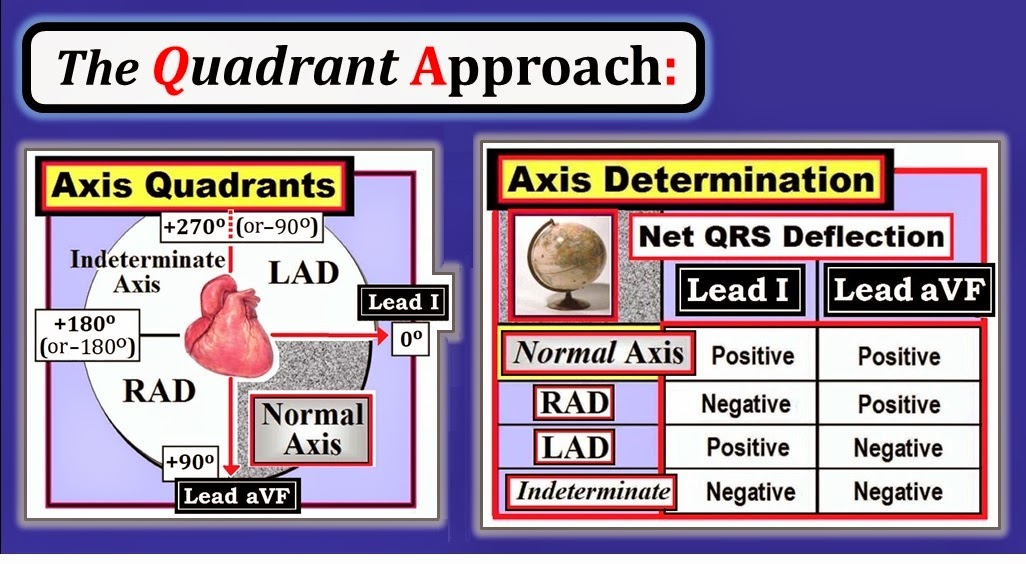Figure-4: The Quadrant Approach for Axis Determination. It is easiest to define Axis as lying within one of four 90-degree Quadrants.

Applying the Quadrant Approach (Example A)
Determining the Quadrant in which the Axis lies is easy — and should take less than 5 seconds to accomplish. Simply use the Table in Figure-4. With no more than a little practice — you’ll find you no longer need to refer to this Table.
• NOTE: By definition, the Axis will be “normal” (ie, within 0 to +90 degrees) — IF the “net” QRS deflection is positive in both lead I and lead aVF.
• But, IF either lead I or lead aVF do not manifest a “net” positive deflection — then there will be Axis “deviation” to one of the other 3 Quadrants (either RAD, LAD or an indeterminate Axis).

Example A: Consider the clinical example in Figure-5. Determine the Quadrant in which the Axis lies. See if you can determine the approximate number of degrees for the Axis.
• NOTE: Since only 2 leads are needed to determine Axis Quadrant  that is all we provide in this and in the schematic examples to follow.Figure-5: Applying the Quadrant Approach. In which Quadrant does the Axis in Example A lie? Estimate the number of degrees for the Axis.

ANSWER to Example A: The Axis is normal (ie, between 0 to +90 degrees). We instantly know this — because the “net” QRS deflection in both lead I and lead aVF in Figure-5 is obviously positive (See Table in Figure-4).
• To facilitate visualization of where the Axis lies — We schematically draw colored arrows on our Axis Quadrant Sketch in Figure-5 that show Lead I (at 0 degreesand Lead aVF (at +90 degrees). The black arrow shows our estimate for the overall Axis.
• Note that the QRS complex in Figure-5 is all positive in both lead I and in lead aVF. IF the R wave was equally tall in these 2 leads — then the Axis would lie midway between them (or at about +45 degrees).
• Since the R wave in lead I of Figure-5 looks to be slightly taller than the R wave in lead aVF (red vs green arrow) — We estimate the axis to be between +30-to-40 degrees (black arrow in Figure).
• Clinically — It is probably enough to simply say that the Axis in Figure-5 is “normal”.

TEST YOURSELF (Example B): What is the Axis?
Determine the Axis Quadrant for Example B (in Figure-6).
• Is the Axis normal? Estimate the number of degrees.Figure-6: Determine the Axis for Example B.

ANSWER to Example B: The Axis in Figure-6 is again normal (ie, between 0 to +90 degrees) — because the QRS is all positive in both lead I and in lead aVF.
• Since the QRS deflection in lead aVF is decidedly more positive — the axis lies closer to aVF (at about +60-75 degrees).
• Clinically — it suffices to say that the Axis is “normal”.
• Remember — All you are doing is approximating! Axis calculation need not be exact — as long as your estimate is within 20-to-30 degrees of the actual axis.

TEST YOURSELF (Example C): What is the Axis?
Determine the Axis Quadrant for Example C (in Figure-7).
• Is the Axis normal? Estimate the number of degrees.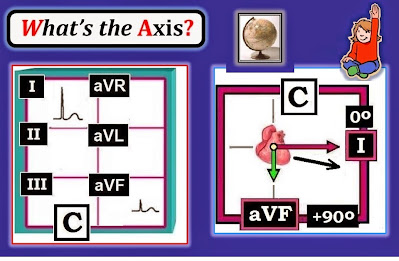Figure-7: Determine the Axis for Example C.

ANSWER to Example C: The Axis in Figure-7 is also normal (ie, between 0 to +90 degrees). We instantly know this — because the QRS complex is all positive in both lead I and in lead aVF.
• Since the R wave in lead I is much more positive than the R wave in lead aVF (red vs green arrow) — We estimate the axis to lie between +10-30 degrees.

TEST YOURSELF (Example D): What is the Axis?
Determine the Axis Quadrant for Example D (in Figure-8).
• Is the Axis normal? Estimate the number of degrees.Figure-8: Determine the Axis for Example D.

ANSWER to Example D: The Axis in Figure-8 is not “normal”. We say this because the “net” QRS deflection is more negative than positive in one of the 2 key leads that are used for axis determination.
• Specifically — the RS complex in lead aVF manifests an S wave that is more negative than the R wave is tall in this lead. This places the Axis in the superior hemisphere (green arrow in Figure-8).
• On the other hand — the QRS complex in lead I is all positive, with a relatively tall R wave. This places the Axis in the left hemisphere (red arrow).
• The presence of a “net” positive deflection in lead I with a “net” negative deflection in lead aVF — defines the Axis as indicating LAD (Left Axis Deviation). That said — the S wave in lead aVF is not all that much deeper than the R wave is tall. We therefore estimate a relatively small amount of LAD (or an Axis of between -10 to -20 degrees).
• Clinically — When the amount of LAD is severe (ie, clearly more negative than seen in Figure-8) — this may indicate a conduction defect known as LAHB (Left Anterior HemiBlock). For the moment — Simply recognize that there is LAD. (We address LAHB below beginning in Figure-11).

===============================
NOTE: The “beauty” of the Quadrant Approach — is that you can tell within seconds IF the axis is normal or not.
• IF the “net” QRS deflection is not positive in both lead I and lead aVF — then the Axis Quadrant is not normal!
===============================

TEST YOURSELF (Example E): What is the Axis?
Determine the Axis Quadrant for Example E (in Figure-9).
• Is the Axis normal? Estimate the number of degrees.Figure-9: Determine the Axis for Example E.

ANSWER to Example E: The Axis in Figure-8 is not “normal” — because the “net” QRS deflection is more negative than positive in one of the 2 key leads.
• Specifically — the RS complex in lead I manifests an S wave that is more negative than the R wave is tall in this lead. This places the Axis in the right hemisphere (red arrow).
• On the other hand — the QRS complex in lead aVF manifests a positive “net” deflection, with an R wave that clearly is taller than the s wave in this lead is deep. This places the Axis in the inferior hemisphere (green arrow).
• The presence of a “net” negative deflection in lead I with a “net” positive deflection in lead aVF — defines the Axis as indicating RAD (Right Axis Deviation). We estimate the Axis to lie between +100 to +120 degrees).
• Clinically — RAD to this degree is most often seen with pulmonary disease and/or an enlarged right ventricle (For more on RVH — See Figure-16).

TEST YOURSELF (Example F): What is the Axis?
Determine the Axis Quadrant for Example F (in Figure-10).
• Is the Axis normal? Estimate the number of degrees.Figure-10: Determine the Axis for Example F.

ANSWER to Example F: The Axis in Figure-10 is not “normal”. We know this — because the “net” QRS deflection is negative in at least one of the 2 key leads used for axis determination.
• In fact — the “net” QRS deflection is negative in both lead I and lead aVF. This places the Axis in the right and superior hemisphere (red and green arrows).
• According to Figure-4 — the presence of a “net” negative deflection in both lead I and lead aVF defines the Axis as indeterminate (ie, lying in the upper right Quadrant).
• NOTE: There is no need to attempt estimating the number of degrees when the Axis is “indeterminate”. As implied in its name — it is virtually impossible to “determine” the specific number of degrees in an indeterminate Axis.
• Clinically — Patients with COPD (Chronic Obstructive Pulmonary Disease) are prone to develop an indeterminate axis (as a result of the large emphysematous chest wall cavity of a patient with COPD).

CLINICAL USE of AXIS Determination
Apply what we’ve covered to determine the Axis for the 12-lead ECG shown in Figure-11. Is the Axis normal?Figure-11: Determine the Axis for this 12-lead ECG. Is the Axis normal?

ANSWER to Figure-11: Although 12 leads are shown here — only 2 of these leads (ie, lead I and lead aVF) are needed to determine the Axis Quadrant. Since the “net” QRS deflection in one of these 2 leads is obviously negative — We know at a glance that the Axis is not “normal”.
• In fact, the QRS deflection in lead aVF is almost entirely negative. This tells us there is marked LAD (Left Axis Deviation) — which leads to discussion of Hemiblocks ...
• NOTE: After we introduce the Hemiblocks — we'll complete this blog post with a few more insights regarding Clinical Use of Axis Determination.

------------------------------------------------------
------------------------------------------------------

HEMIBLOCKS: The Ventricular Conduction System
We illustrate the ventricular conduction system in Figure-12. Note that after the AV Node and Bundle of His — the ventricular conduction system divides into 3 main conduction fascicles. These are: i) The thin right bundle branchii) The relatively thin left anterior hemifascicle, that arises from the short common left bundle branch segment; and, iii) The much thicker and more extensive posterior hemifascicle, which is the other branch arising from the short common left bundle branch segment.
• Failed conduction in the right bundle branch results in RBBB (Right Bundle Branch Block).
• Failed conduction in the common left bundle branch results in LBBB (Left Bundle Branch Block).
• Failed conduction in one of the hemifascicles — results in a Hemiblock (either anterior or posterior, depending on which hemifascicle fails).
• NOTE: LBBB can also occur from failed conduction at a more distal site in the conduction system IF there is block in both the anterior and posterior hemifascicles.Figure-12: The ventricular conduction system. Note how much thicker the left posterior hemifascicle is compared to the anterior hemifascicle and the right bundle branch.

Rapid Diagnosis of the HEMIBLOCKS (LAHB & LPHB)
It is important to appreciate that the illustration of the conduction system in Figure-12 is schematic. In reality — there are millions of fibers in the conduction system, with many potential anatomic variants of the arrangement shown. Nevertheless — the simplified arrangement shown here facilitates discussion.
• As noted — there are 2 major hemifascicles. Failed conduction in one or the other produces one of the 2 types of Hemiblock = i) LAHB (Left Anterior HemiBlock)orii) LPHB (Left Posterior HemiBlock).
• In a resting ECG — the finding of LPHB is rare. In our experience — 98-99% of hemiblocks seen on a resting ECG are LAHB. Reasons why LPHB is rare include: i) The posterior hemifascicle is anatomically much thicker (Figure-12) — therefore more difficult to damage; and, ii) Unlike the anterior hemifascicle, there is a dual blood supply from right and left coronary arteries.
• When LPHB does occur on a resting ECG — it is usually associated with RBBB, and indicates extensive damage (See ECG Blog #30 — for an example of LPHB/RBBB with further discussion).
• Therefore (ie, since ~98-99% of hemiblocks seen on a resting ECG are anterior) — IF a hemiblock is present, it will almost always be LAHB!

• Beyond the Core: A hemiblock and/or bundle branch block may sometimes be seen with fast rhythms. The physiologic reason for this form of aberrant conduction — is that the accelerated heart rate does not allow adequate time for recovery of conduction properties in one or more of the major conduction fascicles. Any of the major conduction blocks may be seen (ie, LAHB; LPHB; LBBB; RBBB — or bifascicular block due to RBBB/LAHB or RBBB/LPHB). In this setting of rate-related aberrant conduction — LPHB aberration is not nearly as rare as in a resting tracing.

KEY Clinical Point:

It's important to appreciate that even expert electrocardiographers do not agree on how to define LAHB. Given this lack of consensus — life is simpler (and equally accurate) — IF you equate pathologic LAD (Left Axis Deviation) = LAHB. Consider the following:
• Some LAD (ie, -10 to -20 degrees) — is common and not necessarily abnormal.
• We define a pathologic LAD — as a left axis more negative than -30 degrees. In Figure-13 — we illustrate how EASY it is to tell IF pathologic LAD is present. This can be done in seconds — You only need to look at lead II.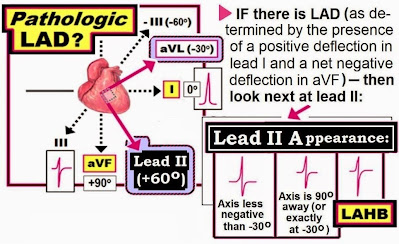Figure-13: Diagnosis of heimblocks is simplified by defining LAHB as a “pathologic” left axis. Assuming the heart lies in the left side of the chest — then IF lead II (at +60 degrees) is isoelectric (equal parts positive and negative) — the Axis lies 90 degrees away (or at -30 degrees). IF lead II is slightly more positive — then the Axis is less than 90 degrees from lead II (ie, less negative than -30 degrees). But IF lead II is more negative than positive — the Axis must lie more than 90 degrees away from lead II (ie, more negative than -30 degrees) — which satisfies our simplified criteria for LAHB.

Summarizing Concept: Quick Diagnosis of LAHB
We have already emphasized how the Axis Quadrant can be determined within seconds by inspection of the net QRS deflection in 2 leads (leads I and aVF).
• Use of a 3rd lead = lead II — allows us to then determine IF a patient with LAD manifests sufficient left axis to qualify for LAHB.
• For practical purposes — No more than these 3 leads are needed when assessing Axis by our Systematic Approach (Figure 14).Figure-14: Use of Lead II to diagnose LAHB. Panels G and H both show LAD (since net QRS deflection is positive in lead I and negative in lead aVF in each example). There is LAHB in Panel H but not in Panel G — because the net QRS deflection is negative in lead II for H (but not in G).

TEST YOURSELF: Is there LAD If so — Is there LAHB?
Put these concepts together in assessing the Axis for the 6 leads shown in Figure-15. We have already seen this tracing (in Figure-11) — and determined that there is marked LAD (net QRS deflection is positive in lead I but very negative in lead aVF).
• How can we tell at a glance IF the amount of LAD in Figure-11 is enough to qualify as LAHB?
• HINT: Which lead should we look at to answer this question?Figure-15: Is the amount of LAD enough to qualify for LAHB? See Answer below.

ANSWER to Figure-15: As emphasized in Figure-13 and Figure-14 — a glance at lead II is all that is needed to confirm that LAHB is present in Figure-15:
• Lead II — is predominantly negative in Figure-15. Therefore — the Axis is more than 90 degrees away from Lead II (ie, more negative than -30 degrees) — therefore LAHB.

===================================
Clinical USE of AXIS:
We conclude today's blog post with illustration of 2 additional ways Axis determination is used clinically.
===================================

Is there RVH?
Consider the 12-lead ECG shown in Figure 16 — obtained from an older adult with heart failure. Sinus rhythm is present. The PR, QRS and QT intervals are all normal.
• Is the Axis normal? If not — Why not?
• Clinically — How does determination of Axis help in our interpretation?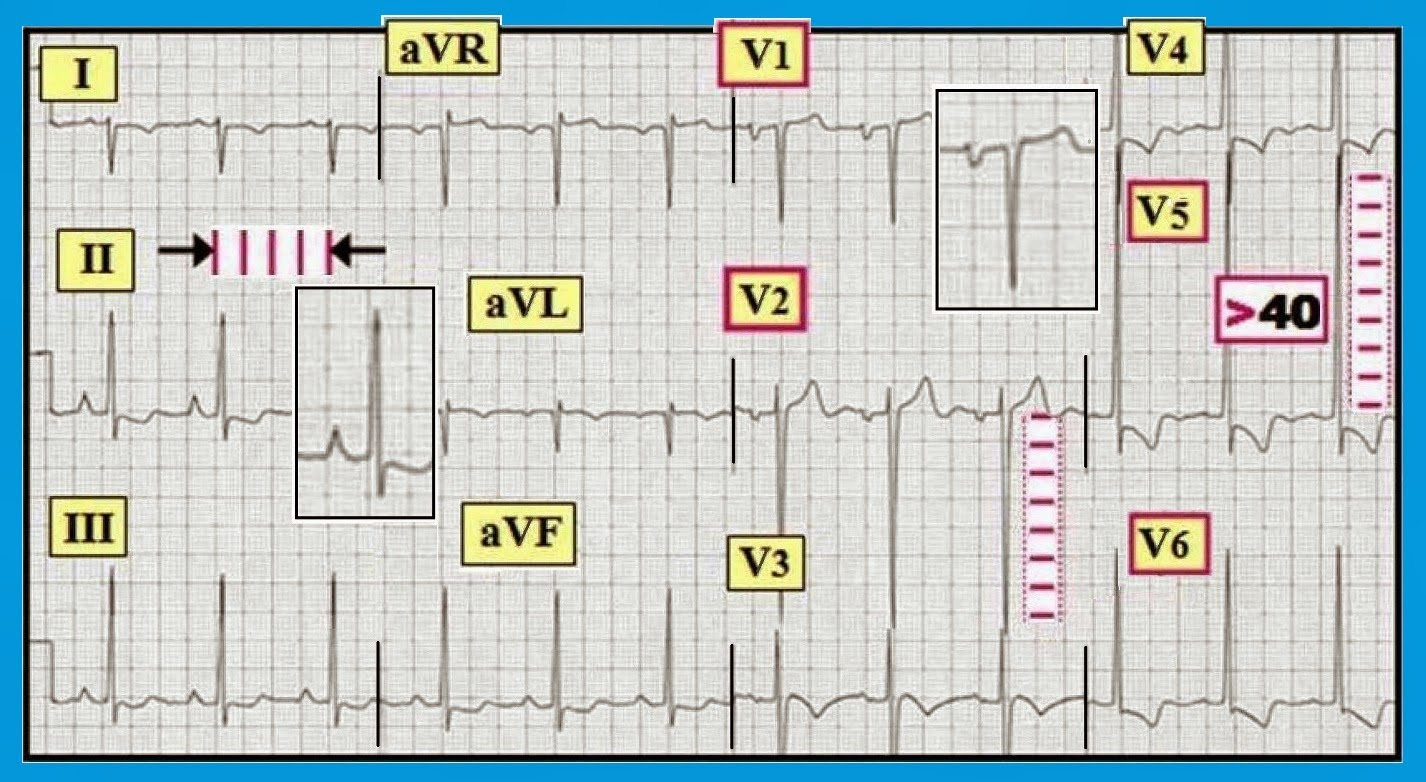Figure-16: ECG obtained from an older adult with heart failure. How does determination of Axis help in our interpretation?

ANSWER to Figure-16: As noted — the rhythm is sinus and all intervals are normal. In addition — there is at least 3 chamber enlargement because: i) Tall, peaked and pointed P waves in lead II = RAA (Right Atrial Abnormality)ii) Deep negative component to the P wave in lead V1 = LAA (Left Atrial Abnormality)and, iii) Voltage criteria for LVH are easily satisfied. ST-T waves are consistent with ischemia and/or “strain” (See our ECG Blog #73Blog #75 and Blog #77 for Review of criteria for LVH; RAA/LAA; and RVH).
• There is marked RAD (Right Axis Deviation) — as determined by the upright QRS complex in lead aVF, in association with the predominantly negative QRS in lead I.
• In the context of an older adult with heart failure and at least 3 chamber enlargement on ECG — the finding of marked RAD + RAA almost certainly indicates that there is also RVH (Right Ventricular Hypertrophy). ST-T wave changes in the inferior leads may in fact reflect right ventricular “strain” from RVH.
• A history of heart failure with evidence of multi-chamber enlargement (in this case of all 4 cardiac chambers) on ECG — is virtually diagnostic of a cardiomyopathy.

Is there VT?
An underappreciated use of frontal plane Axis determination is in the differential diagnosis of a regular WCT (Wide-Complex Tachycardia). I cover this complex topic in detail elsewhere (See ECG Blog #196) — but simply note “the short answer” in the clinical points below:
• Always assume a regular WCT rhythm without normal P waves is VT until proven otherwise. You will be correct >80% of the time (>90% of the time if the patient is middle-aged or older and has underlying heart disease).
• Treat the patient as if the rhythm is VT until proven otherwise.
• Among the numerous diagnostic criteria cited for distinguishing between SVT with either bundle branch block or aberration vs VT — I have found my Simple Rules greatly facilitate the diagnostic process. The likelihood of VT exceeds 90-95% — IF i) The QRS complex is markedly widened and formless; and/or ii) The QRS complex is all negative (or almost all negative) in left-sided lead V6; and/or, iii) There is extreme Axis deviation in the frontal plane (which I define as an Axis in which the QRS complex is all negative in either lead I or in lead aVF).

Q
UESTION:
Which of the above 3 “Simple Rules” are present for the ECG shown in Figure-17? Assume the patient is an older adult with a history of heart disease — and that the patient is hemodynamically stable despite this rhythm.Figure-17: This regular WCT rhythm is from an older adult with heart disease. The patient is hemodynamically stable. Does determination of Axis during this tachycardia assist in rhythm diagnosis?

ANSWER to Figure-17: Realize that statistical odds that this regular WCT is VT in this older adult with heart disease exceed 90% — even before taking a closer look at this tracing! That said — We can increase the likelihood of VT to nearly 100% by noting that all 3 of the above Simple Rules are present!
• The QRS is markedly widened (to at least 0.16 second in some leads) — and “ugly” in appearance (therefore much less likely to reflect bundle branch block or aberrant conduction).
• The QRS complex in lead V6 is entirely negative — which by itself makes the chance of VT nearly 100%!
• There is extreme Axis deviation (ie, the QRS is all negative in lead I) — which also almost by itself makes the chance of VT nearly 100%!

===================================
Relevant ECG Blog Posts to Today's Post: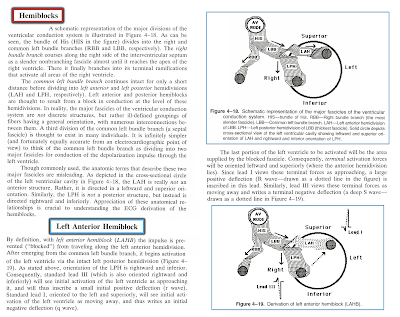Figure-18: Pathophysiology of the Hemiblocks (Overview — LAHB).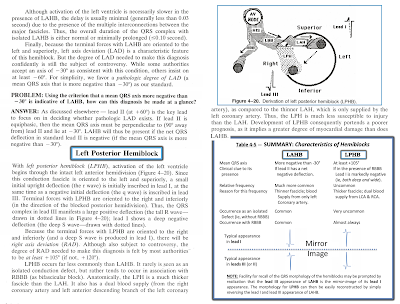Figure-19: Pathophysiology of the Hemiblocks (LPHB — Summarizing Table).